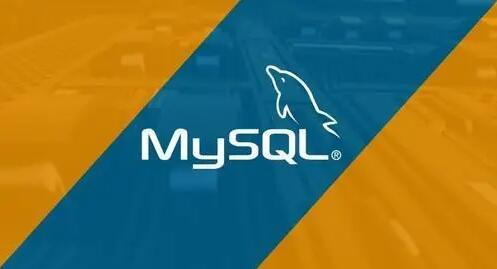mysql批量更新替换删除字段数据UPDATE Content SET [字段]=值 WHERE [字段] is null

UPDATE Content SET [字段]=replace([字段],'原字符串','新字符串')

DELETE FROM Content Where [字段] is null

where解释：

`SELECT field1, field2,...fieldN FROM table_name1, table_name2...[WHERE condition1 [AND [OR]] condition2.....`
• 查询语句中你可以使用一个或者多个表，表之间使用逗号, 分割，并使用WHERE语句来设定查询条件。

• 你可以在 WHERE 子句中指定任何条件。

• 你可以使用 AND 或者 OR 指定一个或多个条件。

• WHERE 子句也可以运用于 SQL 的 DELETE 或者 UPDATE 命令。

• WHERE 子句类似于程序语言中的 if 条件，根据 MySQL 表中的字段值来读取指定的数据。

= 等号，检测两个值是否相等，如果相等返回true (A = B) 返回false。
<>, != 不等于，检测两个值是否相等，如果不相等返回true (A != B) 返回 true。
> 大于号，检测左边的值是否大于右边的值, 如果左边的值大于右边的值返回true (A > B) 返回false。
< 小于号，检测左边的值是否小于右边的值, 如果左边的值小于右边的值返回true (A < B) 返回 true。
>= 大于等于号，检测左边的值是否大于或等于右边的值, 如果左边的值大于或等于右边的值返回true (A >= B) 返回false。
<= 小于等于号，检测左边的值是否小于或等于右边的值, 如果左边的值小于或等于右边的值返回true (A <= B) 返回 true。